## Anomaly Detection with Isolation Forest

### Introduction to Isolation Forest

The isolation forest algorithm  detects anomalies by isolating anomalies from normal points using an ensemble of isolation trees. Each isolation tree is trained for a subset of training observations, sampled without replacements. The algorithm grows an isolation tree by choosing a split variable and split position at random until every observation in a subset lands in a separate leaf node. Anomalies are few and different; therefore, an anomaly lands in a separate leaf node closer to the root node and has a shorter path length (the distance from the root node to the leaf node) than normal points. The algorithm identifies anomalies using anomaly scores defined based on the average path lengths over all isolation trees.

Use the `iforest` function, `IsolationForest` object, and `isanomaly` object function for outlier detection and novelty detection.

• Outlier detection (detecting anomalies in training data) — Detect anomalies in training data by using the `iforest` function. `iforest` builds an `IsolationForest` object and returns anomaly indicators and scores for the training data. For an example, see Detect Outliers.

• Novelty detection (detecting anomalies in new data with uncontaminated training data) — Create an `IsolationForest` object by passing uncontaminated training data (data with no outliers) to `iforest`, and detect anomalies in new data by passing the object and the new data to the object function `isanomaly`. For each observation of the new data, the function finds the average path length to reach a leaf node from the root node in the trained isolation forest, and returns an anomaly indicator and score. For an example, see Detect Novelties.

### Parameters for Isolation Forests

You can specify parameters for the isolation forest algorithm by using the name-value arguments of `iforest`:

• `NumObservationsPerLearner` (number of observations for each isolation tree) — Each isolation tree corresponds to a subset of training observations. For each tree, `iforest` samples `min(N,256)` number of observations from the training data without replacement, where `N` is the number of training observations. The isolation forest algorithm performs well with a small sample size because it helps to detect dense anomalies and anomalies close to normal points. However, you need to experiment with the sample size if `N` is small. For an example, see Examine NumObservationsPerLearner for Small Data.

• `NumLearners` (number of isolation trees) — By default, the `iforest` function grows 100 isolation trees for the isolation forest because the average path lengths usually converge well before growing 100 isolation trees .

### Anomaly Scores

The isolation forest algorithm computes the anomaly score s(x) of an observation x by normalizing the path length h(x):

`$s\left(x\right)={2}^{-\frac{E\left[h\left(x\right)\right]}{c\left(n\right)}},$`

where E[h(x)] is the average path length over all isolation trees in the isolation forest, and c(n) is the average path length of unsuccessful searches in a binary search tree of n observations.

• The score approaches 1 as E[h(x)] approaches 0. Therefore, a score value close to 1 indicates an anomaly.

• The score approaches 0 as E[h(x)] approaches n – 1. Also, the score approaches 0.5 when E[h(x)] approaches c(n). Therefore, a score value smaller than 0.5 and close to 0 indicates a normal point.

### Anomaly Indicators

`iforest` and `isanomaly` identify observations with anomaly scores above the score threshold as anomalies. The functions return a logical vector that has the same length as the input data. A value of logical 1 (`true`) indicates that the corresponding row of the input data is an anomaly.

• `iforest` determines the threshold value (`ScoreThreshold` property value) to detect the specified fraction (`ContaminationFraction` name-value argument) of training observations as anomalies. By default, the function treats all training observations as normal observations.

• `isanomaly` provides the `ScoreThreshold` name-value argument, which you can use to specify the threshold. The default threshold is the value determined when the isolation forest is trained.

### Detect Outliers and Plot Contours of Anomaly Scores

This example uses generated sample data containing outliers. Train an isolation forest model and detect the outliers by using the `iforest` function. Then, compute anomaly scores for the points around the sample data by using the `isanomaly` function, and create a contour plot of the anomaly scores.

Generate Sample Data

Use a Gaussian copula to generate random data points from a bivariate distribution.

```rng("default") rho = [1,0.05;0.05,1]; n = 1000; u = copularnd("Gaussian",rho,n);```

Add noise to 5% of randomly selected observations to make the observations outliers.

```noise = randperm(n,0.05*n); true_tf = false(n,1); true_tf(noise) = true; u(true_tf,1) = u(true_tf,1)*5;```

Train Isolation Forest and Detect Outliers

Train an isolation forest model by using the `iforest` function. Specify the fraction of anomalies in the training observations as 0.05.

`[f,tf,scores] = iforest(u,ContaminationFraction=0.05);`

`f` is an `IsolationForest` object. `iforest` also returns the anomaly indicators (`tf`) and anomaly scores (`scores`) for the training data.

Plot a histogram of the score values. Create a vertical line at the score threshold corresponding to the specified fraction.

```histogram(scores) xline(f.ScoreThreshold,"r-",join(["Threshold" f.ScoreThreshold]))```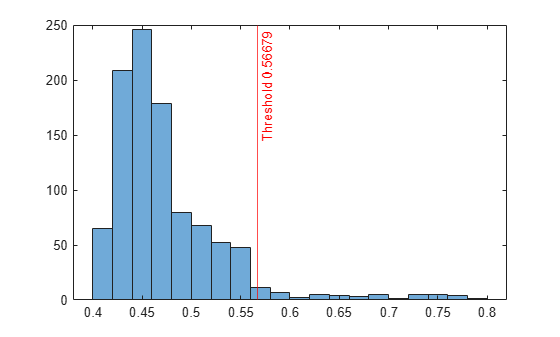Plot Contours of Anomaly Scores

Use the trained isolation forest model and the `isanomaly` function to compute anomaly scores for 2-D grid coordinates around the training observations.

```l1 = linspace(min(u(:,1),[],1),max(u(:,1),[],1)); l2 = linspace(min(u(:,2),[],1),max(u(:,2),[],1)); [X1,X2] = meshgrid(l1,l2); [~,scores_grid] = isanomaly(f,[X1(:),X2(:)]); scores_grid = reshape(scores_grid,size(X1,1),size(X2,2));```

Create a scatter plot of the training observations and a contour plot of the anomaly scores. Flag true outliers and the outliers detected by `iforest`.

```idx = setdiff(1:1000,noise); scatter(u(idx,1),u(idx,2),[],[0.5 0.5 0.5],".") hold on scatter(u(noise,1),u(noise,2),"ro","filled") scatter(u(tf,1),u(tf,2),60,"kx",LineWidth=1) contour(X1,X2,scores_grid,"ShowText","on") legend(["Normal Points" "Outliers" "Detected Outliers"],Location="best") colorbar hold off```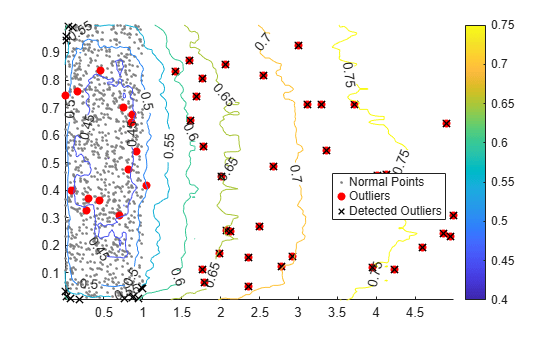Check Performance

Check the performance of `iforest` by plotting a precision-recall curve and computing the area under the curve (AUC) value. Create a `rocmetrics` object. `rocmetrics` computes the false positive rates and the true positive rates (or recall) by default. Specify the `AdditionalMetrics` name-value argument to additionally compute the precision values (or positive predictive values).

`rocObj = rocmetrics(true_tf,scores,true,AdditionalMetrics="PositivePredictiveValue");`

Plot the curve by using the `plot` function of `rocmetrics`. Specify the y-axis metric as precision (or positive predictive value) and the x-axis metric as recall (or true positive rate). Display a filled circle at the model operating point corresponding to `f.ScoreThreshold`. Compute the area under the precision-recall curve using the trapezoidal method of the `trapz` function, and display the value in the legend.

```r = plot(rocObj,YAxisMetric="PositivePredictiveValue",XAxisMetric="TruePositiveRate"); hold on idx = find(rocObj.Metrics.Threshold>=f.ScoreThreshold,1,'last'); scatter(rocObj.Metrics.TruePositiveRate(idx), ... rocObj.Metrics.PositivePredictiveValue(idx), ... [],r.Color,"filled") xyData = rmmissing([r.XData r.YData]); auc = trapz(xyData(:,1),xyData(:,2)); legend(join([r.DisplayName " (AUC = " string(auc) ")"],""),"true Model Operating Point") xlabel("Recall") ylabel("Precision") title("Precision-Recall Curve") hold off```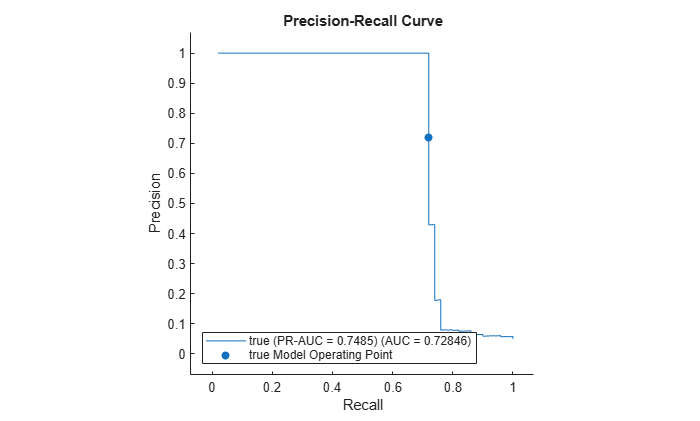### Examine `NumObservationsPerLearner` for Small Data

For each isolation tree, `iforest` samples `min(N,256)` number of observations from the training data without replacement, where `N` is the number of training observations. Keeping the sample size small helps to detect dense anomalies and anomalies close to normal points. However, you need to experiment with the sample size if `N` is small.

This example shows how to train isolation forest models for small data with various sample sizes, create plots of anomaly score values versus sample sizes, and visualize identified anomalies.

`load fisheriris`

The data contains four measurements (sepal length, sepal width, petal length, and petal width) from three species of iris flowers. The matrix `meas` contains all four measurements for 150 flowers.

Train Isolation Forest with Various Sample Sizes

Train isolation forest models with various sample sizes, and obtain anomaly scores for the training observations.

```s = NaN(150,150); rng("default") for i = 3: 150 [~,~,s(:,i)] = iforest(meas,NumObservationsPerLearner=i); end```

Divide the observations into three groups based on the average scores, and create plots of anomaly scores versus sample sizes.

```score_threshold1 = 0.5; score_threshold2 = 0.55; m = mean(s,2,"omitnan"); ind1 = find(m < score_threshold1); ind2 = find(m <= score_threshold2 & m >= score_threshold1); ind3 = find(m > score_threshold2); figure t = tiledlayout(3,1); nexttile plot(s(ind1,:)') title(join(["Observations with average score < " score_threshold1])) nexttile plot(s(ind2,:)') title(join(["Observations with average score in [" ... score_threshold1 " " score_threshold2 "]"])) nexttile plot(s(ind3,:)') title(join(["Observations with average score > " score_threshold2])) xlabel(t,"Number of Observations for Each Tree") ylabel(t,"Anomaly Score")```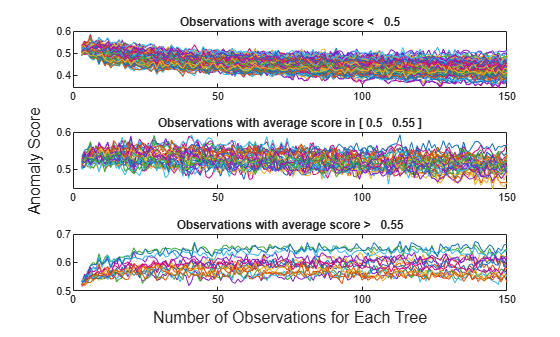The anomaly score decreases as the sample size increases for the observations whose average score values are less than 0.5. For the observations whose average score values are greater than 0.55, the anomaly score increases as the sample size increases and then the score converges roughly when the sample size reaches 50.

Detect anomalies in training observations by using isolation forest models with the sample sizes 50 and 100. Specify the fraction of anomalies in the training observations as 0.05.

```[f1,tf1,scores1] = iforest(meas,NumObservationsPerLearner=50, ... ContaminationFraction=0.05); [f2,tf2,scores2] = iforest(meas,NumObservationsPerLearner=100, ... ContaminationFraction=0.05);```

Display the observation indexes of the anomalies.

`find(tf1)`
```ans = 7×1 14 42 110 118 119 123 132 ```
`find(tf2)`
```ans = 7×1 14 15 16 110 118 119 132 ```

The two isolation forest models have five anomalies in common.

Visualize Anomalies

For the isolation forest model with the sample size 50, visually compare observation values between normal points and anomalies. Create a matrix of grouped histograms and grouped scatter plots for each combination of variables by using the `gplotmatrix` function.

```tf1 = categorical(tf1,[0 1],["Normal Points" "Anomalies"]); predictorNames = ["Sepal Length" "Sepal Width" ... "Petal Length" "Petal Width"]; gplotmatrix(meas,[],tf1,"kr",".x",[],[],[],predictorNames)```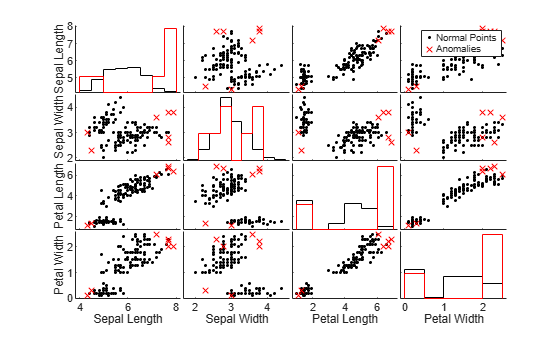For high-dimensional data, you can visualize data by using only the important features. You can also visualize data after reducing the dimension by using t-SNE (t-Distributed Stochastic Neighbor Embedding).

Visualize observation values using the two most important features selected by the `fsulaplacian` function.

`idx = fsulaplacian(meas)`
```idx = 1×4 3 4 1 2 ```
```gscatter(meas(:,idx(1)),meas(:,idx(2)),tf1,"kr",".x",[],"on", ... predictorNames(idx(1)),predictorNames(idx(2)))```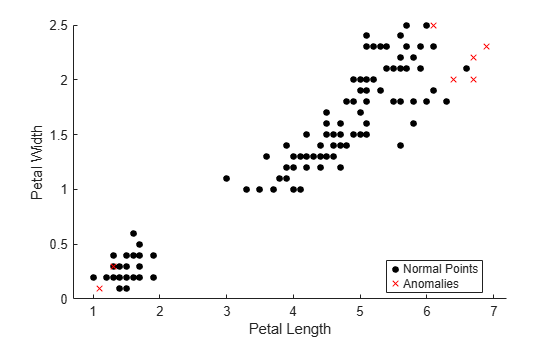Visualize observation values after reducing the dimension by using the `tsne` function.

```Y = tsne(meas); gscatter(Y(:,1),Y(:,2),tf1,"kr",".x")```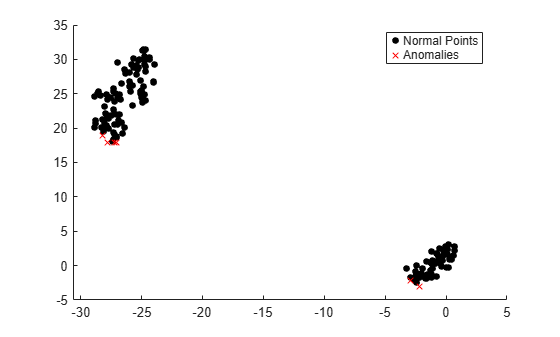Liu, F. T., K. M. Ting, and Z. Zhou. "Isolation Forest," 2008 Eighth IEEE International Conference on Data Mining. Pisa, Italy, 2008, pp. 413-422.